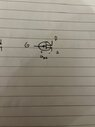# Transistor gate potential

• Engineering
• David331
In summary, this circuit has a JFET stage that is referenced to the negative rail, with output voltage referenced to ground.f

#### David331

Homework Statement
The gate potential
Relevant Equations
?
Hi, not really a homework question just a quick question regarding FET-transistor. If the gate potential is -4V does that mean that in the figure below that uGS is -4V. If not, what does it mean and how do you use it in a bigger circuit problem?

#### Attachments

•8941E6DC-6973-4F2C-80E5-4EFA2FC173E6.jpeg
16.5 KB · Views: 51
It depends, is the -4V specified as a pinch-off voltage or as an operating voltage?
The symbol is for a P-type channel JFET. They are not common.
The negative voltage is an indication that it has a P-channel, because the gate voltage is measured relative to the more positive source, probably operating with the drain negative.
https://en.wikipedia.org/wiki/JFET#Functions

Search for data and circuits that use a 2N5460, 2SJ174 to 2SJ177, 2SJ270, 2SJ271.

For operating a FET the potential difference (voltage) between G and S matters only. Hence, the potential at the gate node is identical to Vgs only in case the source node is at ground potential.

Hmm I am kinda new to transistor so I do not know what pinch-voltage is. The full circuit is as below; the source node is not at ground potential. The task of the assignment is to solve for R4, R5, RS, RD with IDQ, UDSQ, UP , IDSS known and that the gate-potential must be -4V ( and that the FET-amplifier stage must not lower the first amplifier stage with more than 0.5%). So then, how could I use that the gate potential is -4V to get an extra equation to solve for the resistances?

#### Attachments

Notice that the negative supply, -E is at the top of the page;
NPN transistors are most common, this circuit uses PNP transistors.
N-chan JFETs are most common, this uses a P-chan JFET.
This is an exercise in thinking upside down.
The gate potential
Relevant Equations:: ?
Hi, not really a homework question just a quick question regarding FET-transistor.
The task of the assignment is to solve for R4, R5, RS, RD with IDQ, UDSQ, UP , IDSS known and that the gate-potential must be -4V ( and that the FET-amplifier stage must not lower the first amplifier stage with more than 0.5%).
You must know more than you are telling us, or you have taken on a difficult first exercise.
The full circuit is as below; the source node is not at ground potential.
The input to the circuit, Uin(t), is referenced to ground. The first two PNP BJTs make a darlington pair, with output referenced to the negative rail. The final JFET stage has input referenced to negative rail, with output voltage referenced to ground.

You need to study common source JFET amplifiers.
They are based on currents flowing through potential dividers.
https://staff-old.najah.edu/sites/default/files/Chapter 9.pdf

I can show the entire assignment, although it is in Swedish. I forgot to say, but all the other resistances are known, as well as the h- and g- parameters. I can show you how I am thinking. Firstly I analyze the second amplifier stage using DC and I get the circuit below. I then use KVL to get 3 equations. Now I have 3 equations and 5 unknowns. To get one more equation I will rewrite the ciruit with small-signal analysis and use the demand that the second amplifier stage must now lower the first with more than 0.5%, using picture 3. Lastly, I am thinking that the gatepotential set to -4V should somehow give me a fifth equation so the assignment could be solved, or maybe I am completely wrong...
And yes, I do understand that this is a common source JFET, but how could I use that?

#### Attachments

Maybe you do not need to solve a set of equations.
You might walk through the design.
R4 and R5 make a potential divider.
Select values that give Vg = -4 V.
Pick Rs to give low output impedance relative to Rload.

Hmm I think we are not allowed to pick Rs as you say, even though if it in practice works this way. We "must" solve equations in this way, as the examinator did. But now that you say it, I see that I can relate R4 and R5 with Vg, but how do I do it?

It is a potential divider.
i = -E / ( R4 + R5 )
Vg = i * R5

Oh, of course, thanks for the help. I did not know what potential divider was in Swedish...